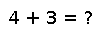# polygamma

polygamma(m, x)

Compute the polygamma function of order `m` of argument `x` (the `(m+1)th` derivative of the logarithm of `gamma(x)`)

## Examples

Checking you are not a robot: